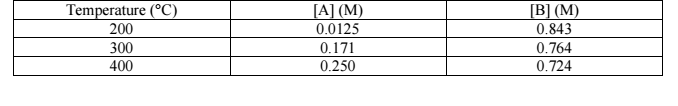# Problem: Consider the following reaction at equilibrium: A (g) ⟺ 2B (g)From the data shown below, determine if the forward reaction is endothermic or exothermic - be sure to explain how you came to that conclusion.

###### FREE Expert Solution
97% (404 ratings)
###### Problem Details

Consider the following reaction at equilibrium: A (g) ⟺ 2B (g)

From the data shown below, determine if the forward reaction is endothermic or exothermic - be sure to explain how you came to that conclusion.What scientific concept do you need to know in order to solve this problem?

Our tutors have indicated that to solve this problem you will need to apply the Le Chatelier's Principle concept. You can view video lessons to learn Le Chatelier's Principle . Or if you need more Le Chatelier's Principle practice, you can also practice Le Chatelier's Principle practice problems.

What is the difficulty of this problem?

Our tutors rated the difficulty ofConsider the following reaction at equilibrium: A (g) ⟺ 2B (...as medium difficulty.

How long does this problem take to solve?

Our expert Chemistry tutor, Jules took 2 minutes and 51 seconds to solve this problem. You can follow their steps in the video explanation above.

What professor is this problem relevant for?

Based on our data, we think this problem is relevant for Professor Payne's class at SPELMAN.